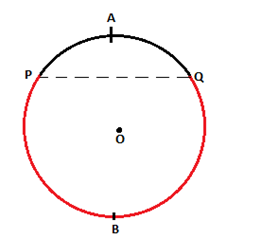# Arc of a Circle Calculator

The Arc of a Circle an online tool which shows Arc of a Circle for the given input. Byju's Arc of a Circle is a tool which makes calculations very simple and interesting. If an input is given then it can easily show the result for the given number. Byju’s arc of a circle calculator is an amazing online tool which assists in determining the length of an arc of circle if the radius and the angle subtended by the arc at the center are given. The working of this calculator can be explained as follows: A set of all points in a plane which are equally spaced from a fixed point is known as a circle. The perimeter of the circle is known as its circumference. A chord of circle is the line segment joining any two points on the circumference of the circle. An arc of a circle is a part of the circumference. Arc is a continuous piece of the circle. In the figure given below , the region of circumference PAQ and PBQ represent two arcs of the circle.The arc PAQ is known as the minor arc and arc PBQ is the major arc. The length of an arc of a circle which subtends an angle of ‘Ɵ’ (in degrees) and has a radius of ‘r’ units is given as: Arc length = r × Ɵ ×(π/180) To find the length of arc of any circle , simply input the radius and the angle subtended by it at the center in degrees in the provided box. You are now just a click away from the result. Thus , Byju's arc of a circle calculator is a tool which makes tough calculations easy and helps us solve difficult problems in span of seconds. Enjoy practicing other related concepts with Byju’s.